Thanks to the Bok Center for Teaching and Learning
(and especially Diane Andronica) for producing this movie.

# Pecha-Kucha

## ArXiv Submission , March 24, 2014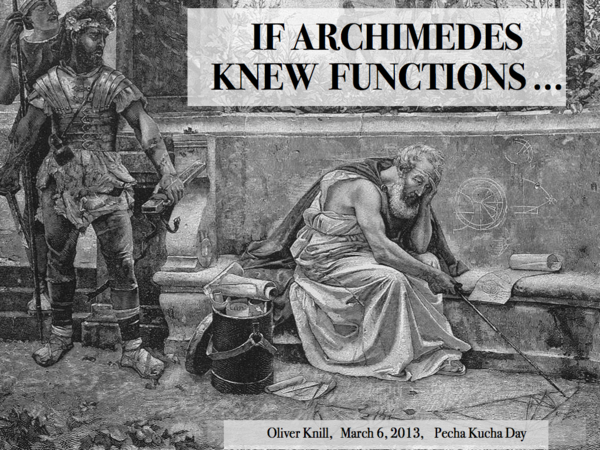What if Archimedes would have known the concept of a function? The following story borrows from classes taught to students in singular variable calculus and at the extension school. The mathematics is not new but illustrates part of what one calls ``quantum calculus" or ``calculus without limits". Remarks: Quantum calculus comes in different flavors. A book with that title was written by Katz and Cheng. The field has connections with numerical analysis, combinatorics and even number theory. The calculus of finite differences was formalized by George Boole but is much older. Even Archimedes and Euler thought about calculus that way. It also has connections with nonstandard analysis. In Math 1a or the extension school, I spent 2 hours each semester with such material. Also, for the last 10 years, a small slide show at the end of the semester in multivariable calculus featured something about beyond calculus. You can click on a slide to the left to see it larger.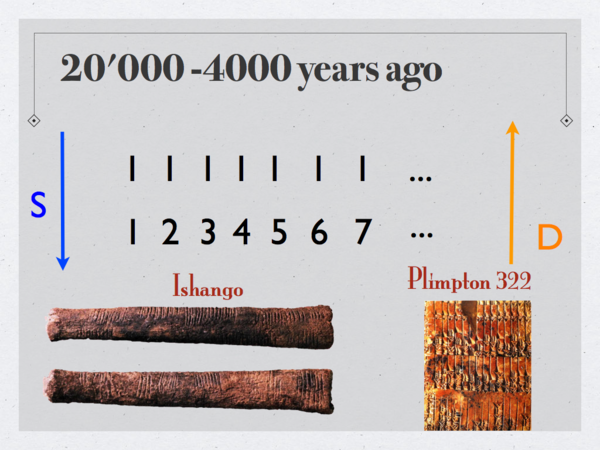About 20 thousand years ago, mathematicians represented numbers as marks on bones. I want you to see this as the constant function 1. Summing up the function gives the concept of number. The number 4 for example is 1+1+1+1. Taking differences brings us back: 4-3=1. Remarks: The Ishango bone displayed in the slide is now believed to be about 20'000 years old. The number systems have appeared in the next couple of thousand years, independently in different places. They were definitely in place 4000 BC, because we have Carbon dated Clay tablets featuring numbers. Its fun to make your own Clay tablets: Either on clay or on chewing gum.When summing up the previous function f(x)=x, we get triangular numbers. They represent the area of triangles. Gauss got a formula for them as a school kid. Summing them up gives tetrahedral numbers, which represent volumes. Integration is summation, differentiation is taking differences. Remarks: The numbers [x]n/n! are examples of Polytopic numbers, numbers which represent patterns. Examples are Triangular, Tetrahedral or Pentatopic numbers. Since we use the forward difference f(x+1)-f(x) and start summing from 0, our formulas are shifted. For example, the triangular numbers are traditionally labeled n(n+1)/2, while we write n(n-1)/2. We can think about the new functions [x]n as a new basis in the linear space of polynomials.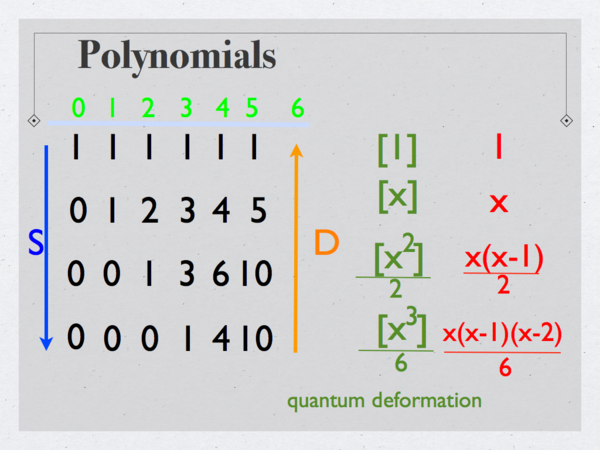The polynomials appear as part of the Pascal triangle. We see that the summation process is the inverse of the difference process. We use the notation [x]n/n! for the function in the nth row. Remarks: The renaming idea [x]n is part of quantum calculus. It is more natural when formulated in a non-commutative algebra, a crossed product of the commutative algebra we are familiar with. The commutative X=C* algebra C(R) of all continuous real functions encodes the topology of the real numbers. If s(x)=x+1 is translation, we can look at the algebra of operators on H=L2(R) generated by X and the translation operator s. With [x]= x s*, the operators [x]n are the multiplication operators generated by the polynomials of the slide. The derivative Df be written as the commutator Df = [s,f]. In quantum mechanics, functions are treated as operators. The deformed algebra is no more commutative: with Qf(x)=x s* satisfying Qn=[xn] and Pf=iDf the anti-commutation relations [Q,P]=QP-PQ=i hold. Hence the name ``quantum calculus".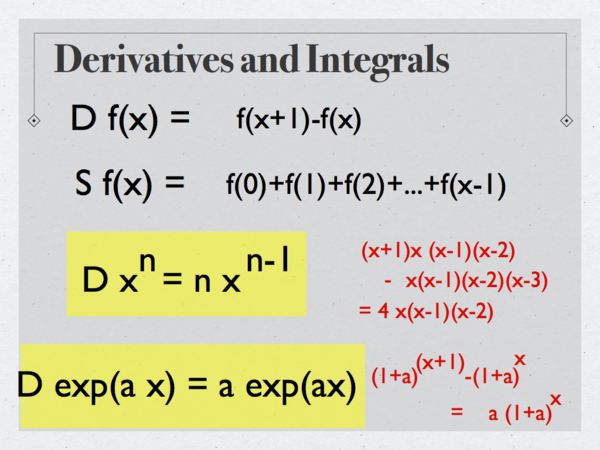With the difference operation D and the summation operation S, we already get an important formula. It tells that the derivative of x to the n is n times x to the n-1. Lets also introduce the function exp(a x) = (1+a) to the power x. This is the compound interest formula. We check that its derivative is a constant times the function itself. Remarks: The deformed exponential is just a rescaled exponential with base (1+a). Writing it as a compound interest formula allows to see the formula exp'(ax)=a exp(ax) as the property that the bank pays you a multiple a exp(ax) to your fortune exp(ax). The compound interest formula appears in this movie. We will below look at the more general exponential exph(x) = (1+a h)x/h which is the exponential exp(a x) to the "Planck constant" h. If h goes to zero, we are lead to the standard exponential function exp(x). One can establish the limit because exph(a x) ≤ exp(a x) ≤ (1+a h) exph(a x).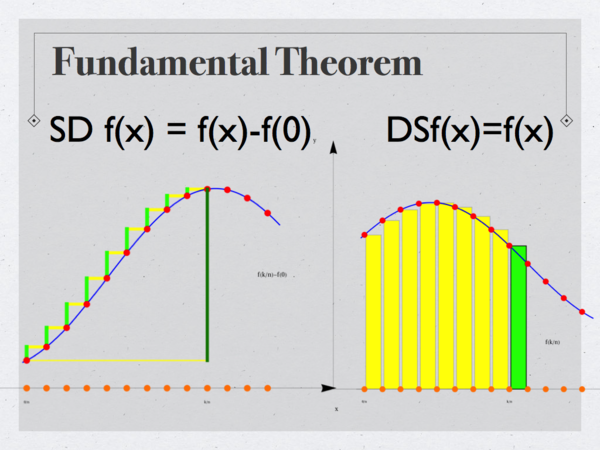The heart of calculus is the fundamental theorem of calculus. If we take the sum of the differences, we get the difference of f(x) minus f(0). If we take the difference of the sum, we get the function f(x). The pictures pretty much prove this without words. These formulas are true for any function. They do not even have to be continuous. Remarks: Even so the notation D is simpler than d/dx and S is simpler than the integral sign, it is the language with D,S which makes the theorem look unfamiliar. Notation is very important in mathematics. Unfamiliar notation can lead to rejection. One of the main points to be made here is that we do not have to change the language. We can leave all calculus books as they are. Just look at the content in new eyes. The talk is advertisement for the calculus we know, teach and cherish. It is a much richer theory than anticipated.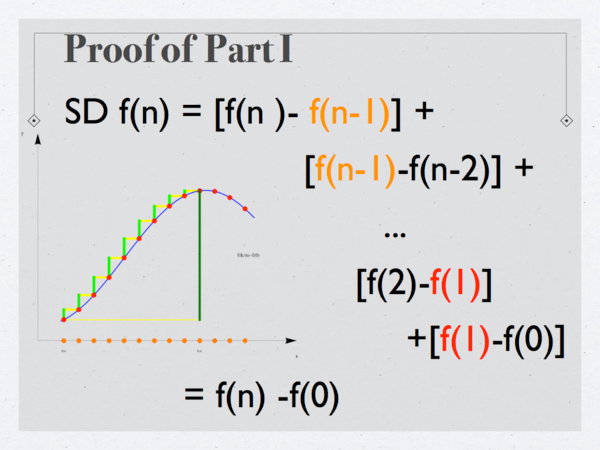The proof of part I shows a telescopic sum. The cancellations are at the core of all fundamental theorems. They appear also in multivariable calculus in differential geometry and beyond. Remarks: It is refreshing that one can show the proof of the fundamental theorem of calculus early in a course. Traditionally, it takes much longer until one reaches the point. Students have here only been puzzled about the fact that the result holds only for x=nh and not in general. It would already here mathematically make more sense to talk about a result on a finite linear graph but that would increase mentally the distance to the traditional calculus. Here is an article of Bressoud on "Historical Reflections on Teaching the fundamental Theorem of Integral Calculus". Bressoud tells that "there is a fundamental problem with the statement of the FTC and that only a few students understand it". I hope this Pecha-Kucha can help to see this important theorem from a different perspective.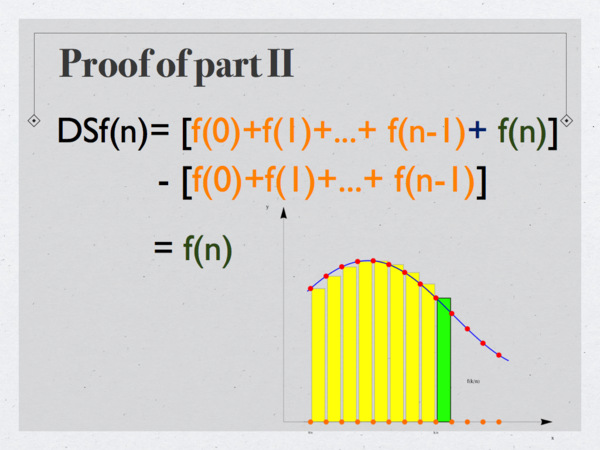The proof of the second part is even simpler. The entire proof can be redone, when the step size h=1 is replaced by a positive h. The fundamental theorem allows us to solve a difficult problem: summation is global and hard, differences is local and easy. By combining the two we can make the hard stuff easy. Remarks: This is the main point of calculus. Integration is difficult, Differentiation is easy. Having them linked makes the hard things easy. At least part of it. It is the main reason why calculus is so effective. These ideas go over to the discrete. Seeing the fundamental idea first in a discrete setup can help to take the limit when h goes to zero.We can adapt the step size h from 1 to become any positive number h. The traditional fundamental theorem of calculus is stated here also in the notation of Leibniz. In classical calculus we would take the limit h to 0, but we do not go that way in this talk. Remarks: When teaching this, students feel a slight unease with the additional variable h. For mathematicians it is natural to have free parameters, for students who have little exposure to mathematics, it is difficult to distinguish between the variable x and h. This is the main reason why in this talk, I mostly stuck to the case h=1. In some sense, the limit h to 0 is an idealization. This is why the limit h going to 0 is so desirable. But we pay a prize: the class of function we can deal with, is much smaller. I myself like to see traditional calculus as a limiting case of a larger theory. The limit h to zero is elegant and clean. But it takes a considerable effort at first to learn what a limit is. Everybody who teaches the subject can confirm the principle that things which were historically hard to find are also harder to master. Nature might have given up on it early on: 10-37 seconds after the big bang: Darn, lets just do it without limits ...!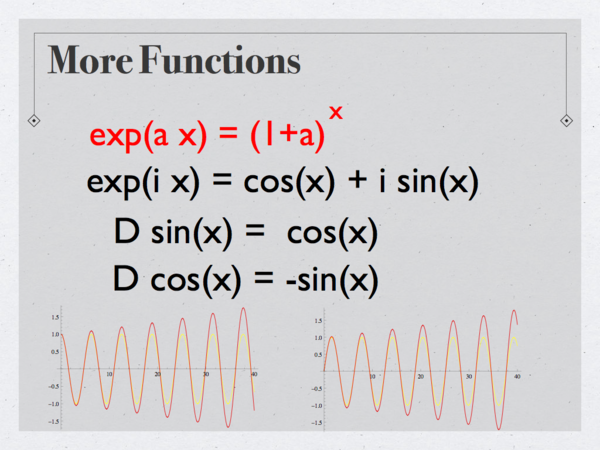We can define cosine and sine by using the exponential, where the interest rate is the square root of -1. These deformed trig functions have the property that the discrete derivatives satisfy the familiar formula as in the books. These functions are close to the trig functions we know if h is small. Remarks: This is by far the most elegant way to introduce trig functions also in the continuum. Unfortunately, due to lack of exposure to complex numbers, it is rarely done. The Planck constant is h=6.626068 . 10-34 m2 kg/s. The new functions (1+i h a )(x/h) = cos(a x) + i sin(a x) are then very close to the traditional cos and sin functions. The functions are not periodic but a growth of amplitude is only seen if x a2 is of the order 1/h. It needs even for X rays astronomical travel distances to see the differences.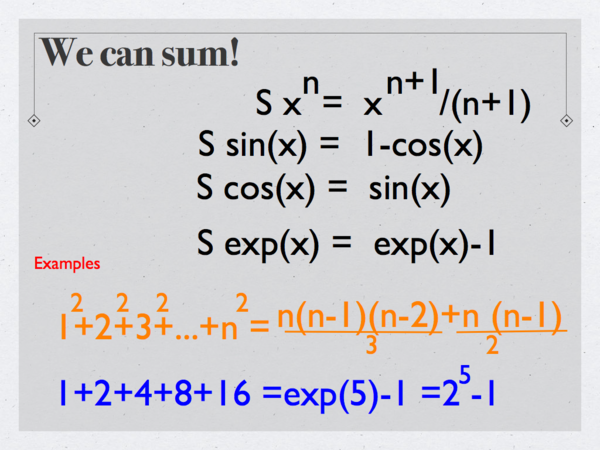The fundamental theorem of calculus is fantastic because it allows us to sum things which we could not sum before. Here is an example: since we know how to sum up the deformed polynomials, we can find formulas for the sum of the old squares. We just have to write the old squares in terms of the new squares which we know how to integrate. Remarks: This leads to a bunch of interesting exercises. For example, because x2 = [x2] + [x], we have S x2 = S [x2] + S [x] = [x3]/3 + [x2]/2 we get a formula for the sum of the first n-1 squares. Again we have to recall that we sum from 0 to n-1 and not from 1 to n. By the way: this had been a 'back and forth' in the early lesson planning. I had started summing from 1 to n and using the backwards difference Df(x) = f(x)-f(x-1). The main reason to stick to the forward difference and so to polynomials like [x]9 = x (x-h) (x-2h)...(x-8h) was that we are more familiar with the difference quotient [f(x+h)-f(x)]/h and also with left Riemann sums. The transition to the traditional calculus becomes easier because difference quotients and Riemann sums are usually written that way.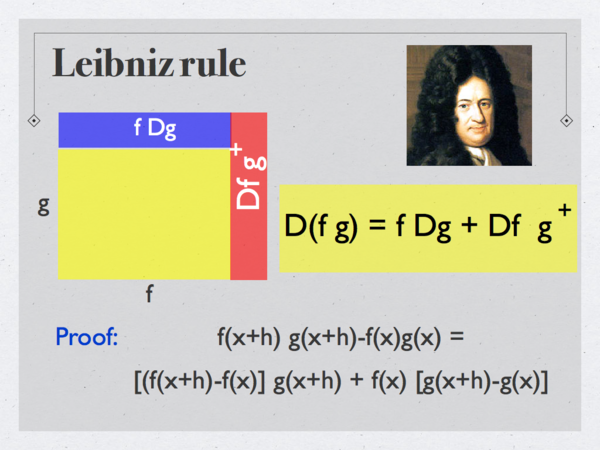We need rules of differentiation and integration. Reversing rules for differentiation leads to rules of integration. The Leibniz rule is true for any function, continuous or not. Remarks: The integration rule analogue to integration by parts is called Abel summation S(f g) = S(f) g - S (Sf Dg) which is important when studying Dirichlet series. A handout [PDF]. I myself used Abel summation in this project. The Leibniz formula has a slight asymmetry. The expansion of the rectangle has two main effects f Dg and Df g, but there is an additional small part Df Dg. This is why we have D(f g) = f Dg + Df g+. The formula becomes more natural when working in the non commutative algebra mentioned before: if Df=[s,f], then D(fg) = f Dg + Df g because the translation operator s took care of the additional shift: (Df) g =(f(x+1)-f(x))g(x+1). The algebra picture also explains why [x]n [x]m is not [x]n+m because also the multiplication operation is deformed in that algebra. In the noncommutative algebra we have (x s*)n (x s*)m = (x s*)n+m. While the algebra deformation is natural for mathematicians, it can not be used in calculus courses. This can be a reason why it appears strange at first, like quantum mechanics in general.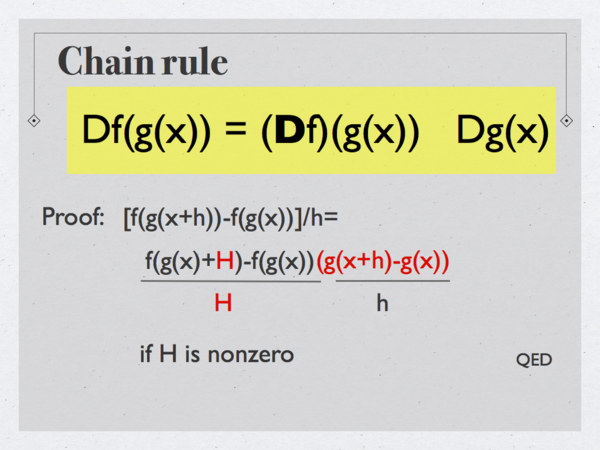The chain rule also looks the same. The formula is exact and holds for all functions. Writing down this formula convinces you that the chain rule is correct. The only thing which needs to be done in a traditional course is to take the limit. Remarks: Most calculus textbooks prove the chain rule using linearization: first verify it for linear functions then argue that the linear part dominates in the limit. [Update Dec 2013: The proof with the limit (Monthly, December 2013) by Haryono Tandra]. Reversing the chain rule leads to the integration tool substitution. Substitution is more tricky here because as we see different step sizes h and H in the chain rule. This discretization issue looks more serious than it is. First of all, compositions of functions like sin(sin(x)) are remarkably sparse in physics. Of course, we encounter functions like sin(k x) but they should be seen as fundamental functions and defined like sin(k h) = im (1+k h i)(x/h). This is by the way different from (1+h i)(k x)/h.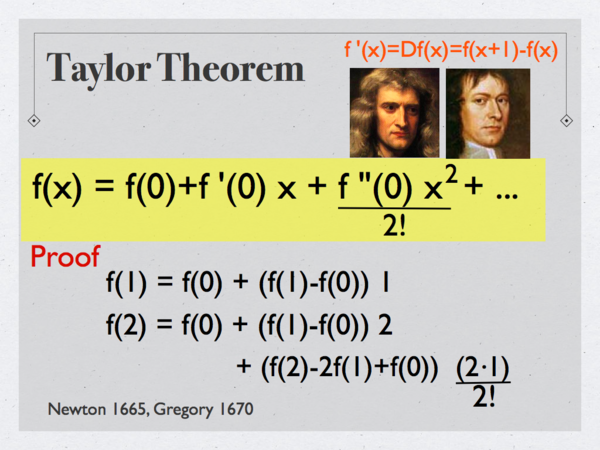Also the Taylor theorem remains true. The formula has been discovered by Newton and Gregory. Written like this, using the deformed functions, it is the familiar formula we know. We can expand any function, continuous or not, when knowing the derivatives at a point. It is a fantastic result. You see the start of the proof. It is a nice exercise to make the induction step. Remarks: James Gregory was a Scottish mathematician who was born in 1638 and died early in 1675. He has become immortal in the Newton-Gregory interpolation formula. Despite the fact that he gave the first proof of the fundamental theorem of calculus, he is largely unknown. As a contemporary of Newton, he was also in the shadow of Newton. It is interesting how our value system has changed. ``Concepts" are now considered less important than proofs. Gregory would be a star mathematician today. The Taylor theorem can be proven by induction. As in the continuum, the discrete Taylor theorem can also be proven with PDE methods: f(x+t) solves the transport equation Dt f = D f, where Dtf(x,t) = f(x,t+1)-f(x,t) so that f(0,t) = [exp(D t) f](0) = f(0) + Df(0)/1! + D2f(0)/2! + ...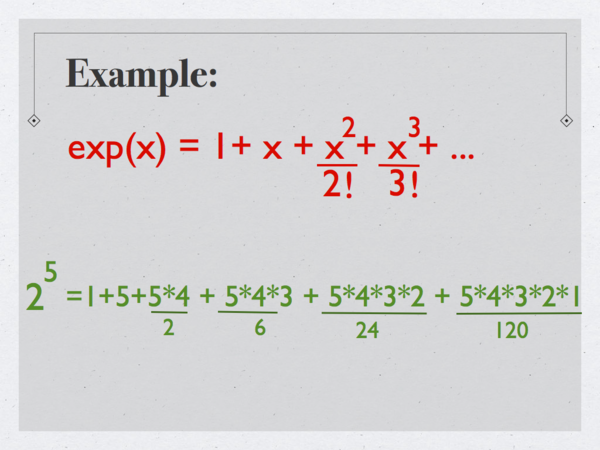Here is an example, where we expand the exponential function and write it as as a sum of powers. Of course, all the functions are deformed functions. The exponential function as well as the polynomials xn were newly defined. We see what the formula means for x=5. Remarks: It is interesting that Taylors theorem has such an arithmetic incarnation. Usually, in numerical analysis text, this Newton-Gregory result is treated with undeformed functions, which looks more complicated. The example on this slide is of course just a basic property of the Pascal triangle. The identity given in the second line can be rewritten as 32 = 1+5+10+10+5+1 which in general tells that if we sum the n'th row of the Pascal triangle we get 2n. Combinatorially, this means that the set of all subsets can be counted by grouping sets of fixed cardinality. It is amusing to see this as a Taylor formula. But it is more than that: it illustrates again an important point I wanted to make: we can not truly appreciate combinatorics if we do not know calculus.Taylor's theorem is useful for data interpolation. We can avoid linear algebra and directly write down a polynomial which fits the data. I fitted here the Dow Jones data of the last 20 years with a Taylor polynomial. This is faster than data fitting using linear algebra. Remarks: When fitting the data, the interpolating function starts to oscillate a lot near the ends. But thats not the deficit of the method. If we fit n data points with a polynomial of degree n-1, then we get a unique solution. It is the same solution as the Taylor theorem gives. The proof of the theorem is a simple induction step, using a property of the Pascal triangle. Assume, we know f(x). Now apply D, to get Df(x). We can now get f(x+1) = f(x) + Df(x). Adding the terms requires a property of the Pascal triangle.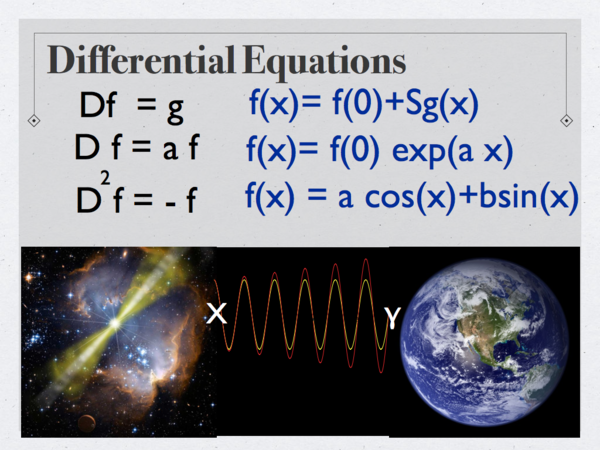We can also solve differential equations. We can use the same formulas as you see in books. We deform the operators and functions so that everything stays the same. Difference equations can be solved with the same formulas as differential equations. Remarks: To make the theory stronger, we need also to deform the log, as well as rational functions. This is possible in a way so that all the formulas we know in classical calculus holds: first define the log as the inverse of exp. Then define [x]-1 = D log(x) and [x]-n = D [x]1-n)/(1-n). Now D xn = n xn-1 holds for all integers. We can continue like that and define sqrt(x) as the inverse of x2 and then the x-1/2 as 2D sqrt(x). It is calculus which holds everything together.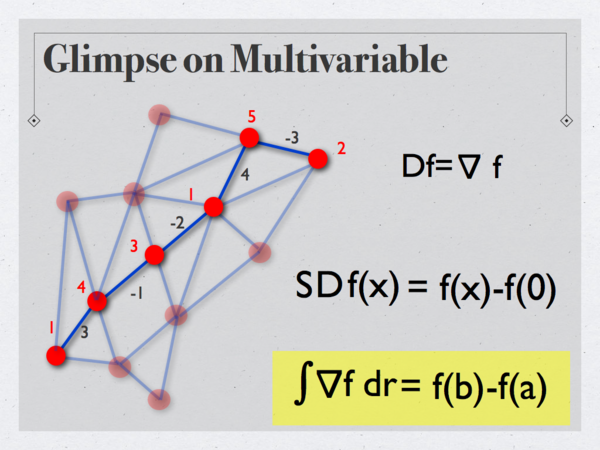Multivariable calculus works too. Space takes the form of a graph. Scalar functions are functions on vertices, Vector fields are signed functions on oriented edges. The gradient of a function is a vector field which is the difference of function values. If we integrate the gradient of a function along a closed path, we get the difference between the potential values at the end points. This is the fundamental theorem of line integrals. Remarks: This result is is also in the continuum the easiest version of Stokes theorem. Technically, one should talk about one forms instead of vector fields. The one forms are anti-commutative functions on edges. Here [ArXiv] is an exhibit of three theorems (Green-Stokes, Gauss-Bonnet,Poincaré-Hopf), where everything is defined and proven on two pages.Stokes theorem holds for a graph for which the boundary is a graph too. Here we see an example of a "surface", which is a union of triangles. The curl of a vector field F is a function on triangles is defined as the sum of vector fields along the boundary. Since the terms on edges in the intersection of triangles cancel, only the line integral along the boundary survives. Remarks: This result is old. It certainly seems have been known to Kirchhoff in 1850. Discrete versions of Stokes pop up again and again over time. It must have been Poincaré who first fully understood the Stokes theorem in all dimensions and in the discrete, when developing algebraic topology. He introduced chains because unlike graphs, chains are closed under boundary operation. This is a major reason, algebraic topologists use it even so graphs are more intuitive. One can for any graph define a discrete notion of differential form as well as an exterior derivative. The boundary of graph is in general only a chain. For geometric graphs like surfaces made up of triangles, the boundary is a union of closed paths and Stokes theorem looks like the Stokes theorem we teach.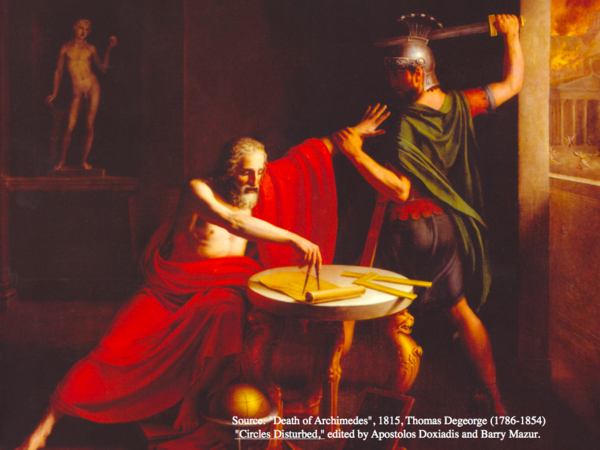Could Archimedes have discovered the fundamental theorem of calculus? His intellectual achievements surpass what we have seen here by far. Yes, he could have done it. If he would not have been given the sword, but the concept of a function. Remarks: Both the precise concept of limit as well as the concept of functions had been missing. While the concept of limit is more subtle, the concept of function is easier. The basic ideas of calculus can be explained without limits. Since the ideas of calculus go over so nicely to the discrete, I believe that calculus is an important subject to teach. It is not only a benchmark and a prototype theory, a computer scientist who has a solid understanding of calculus or even differential topology can also work much better in the discrete. To walk the talk: Here is the source code of a program in computer vision (written totally from scratch) which takes a movie and finds and tracks features like corners. There is a lot of calculus used inside.

This was a talk given on March 6 at a Pecha-Kucha event at the Math department, organized by Sarah Koch and Curt McMullen. Thanks to both for running that interesting experiment.